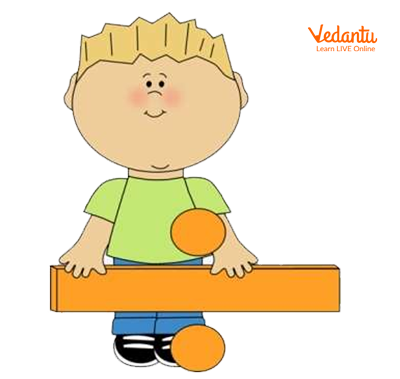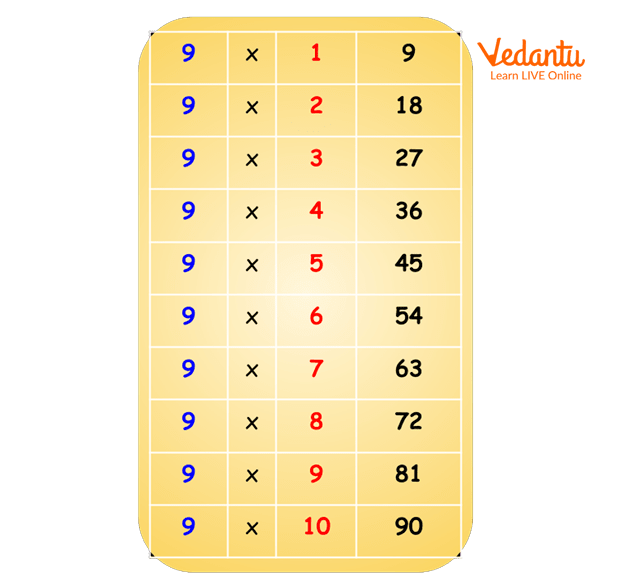Courses
Courses for Kids
Free study material
Free LIVE classes
More

# Test of Divisibility of 9LIVE
Join Vedantu’s FREE Mastercalss

## Introduction to Divisibility

What if I tell you that you can find if a number as big as 34117326 is divisible by 9 without doing the tedious process of long division? You might be thinking that it is some sort of magic trick when in reality it is one of the amazingly logical yet simple techniques of mathematics. If you’ve guessed it already, you’re right! We’re talking about the “Test of Divisibility of 9” Let’s get started on how this works.Divisibility Test for 9

To test the divisibility of 9, we need to know its divisibility rule.in Mathematics are a set of rules specific to each number which help us check whether that number is divisible by a particular number or not, without performing long division. Some common divisibility tests are for numbers 2 to 20.

## Important Things to Recall

• Good old addition (if you’re telling me 2+3 = 7, you first need to work on that).

• Multiplication table of 9 or (once you know 9 times 10 is 90, you are good to go!)Multiplication Table of 9

## Rule of Divisibility for 9

The rule of divisibility for 9 basically goes like this: “A number is divisible by 9 if the sum of all the digits present in the number is divisible by 9”.

To break down this complex-sounding rule, all you have to do is add up all the digits present in the number and see if that sum is divisible by 9.

Now, let’s check if this trick works with an example.

Let’s try it with 198

First, add all the digits in this number.

1 + 9 + 8 = 18

Now all you have to do to check is if 18 is divisible by 9, and the answer to that is, yes!

Hence, 198 is divisible by 9 too!

To get a better hang of this rule of divisibility, let’s practice with a few examples

## Solved Examples

Q1. Check if 171 is divisible by 9.

Ans: 1+7+1=Z

9 is divisible by 9

Hence, 171 is divisible by 9

Q2. Check if 786 is divisible by 9.

Ans: 7+8+6=21

21 is not divisible by 9

Hence 786 is NOT divisible by 9

Q3. Check if 34117326 is divisible by 9.

Ans: 3+4+1+1+7+3+2+6=27

27 is divisible by 9

Hence 34117326 is also divisible by 9

“Practice makes a man perfect”. Now, let’s solve some more numbers to understand and master divisibility by 9.

## Practice Problems

1. Is 725 divisible by 9? (Ans: Yes)

2. Is 5670 divisible by 9? (Ans: No)

3. Is 19997 divisible by 9? (Ans: Yes)

## Summary

Let’s briefly summarise what we’ve learned up until now.

• Divisibility by 9 can be found by adding the digits present in the number.

• If the sum obtained is divisible by 9 (multiple of 9) the number is divisible by 9.

• If the sum obtained is not divisible by (not a multiple of 9) the number is not divisible by 9.

Last updated date: 30th Sep 2023
Total views: 126k
Views today: 3.26k

## FAQs on Test of Divisibility of 9

1. Is there an exception or limit for the rule of divisibility of 9?

No, the rule applies for all the numbers ranging from 2-digit to 7, and 8-digit numbers. Maths has an amazing sequence of logic! This rule holds good for any small to big numbers. All you have to do is simply add the digits of the given number and check if the obtained sum is a multiple of 9.

2. Does this same rule apply to any other divisibility rule?

A similar rule of the sum of digits applies for the divisibility of 3 and partly for the divisibility of 6.

If the sum of the digits present in the given number is a multiple of 3 then the given number is also divisible by 3.

Likewise, a similar rule can be applied for 6 as well. The sum of the digits of a given number must be divisible by both 2 and 3 to be divisible by 6.

3. Is it necessary to know the multiplication table of 9 to master divisibility by 9?

Yes, a multiplication table of 9 is rather easy. Remembering the table till 10 makes the whole process less time-consuming and easy. So, it is necessary to remember the table of 9. Several tips are available online to easily remember the table of 9 like the one illustrated below:

All you have to do is write down 0-9 vertically for the left digit and 9-0 vertically right next to it for the previously written digit to get the multiples of 9!

Look at this:

0 9

1 8

2 7

3 6

4 5

5 4

6 3

7 2

8 1

9 0